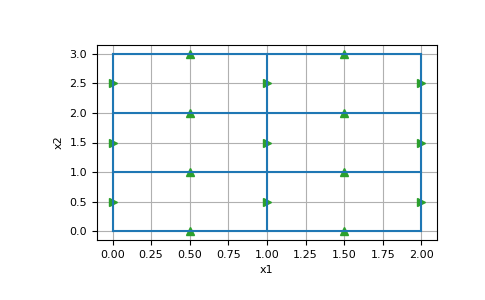# discretize.TreeMesh.n_faces_per_direction¶

property TreeMesh.n_faces_per_direction

The number of faces in each axis direction

This property returns a tuple with the number of faces in each axis direction of the mesh. For a 3D mesh, n_faces_per_direction would return a tuple of the form (nFx, nFy, nFz). Thus the length of the tuple depends on the dimension of the mesh.

Returns
(`dim`) `tuple` `of` `int`

Number of faces in each axis direction

Notes

Property also accessible as using the shorthand vnF

Examples

```>>> import discretize
>>> import numpy as np
>>> import matplotlib.pyplot as plt
>>> M = discretize.TensorMesh([np.ones(n) for n in [2,3]])
>>> M.plot_grid(faces=True)
>>> plt.show()
```